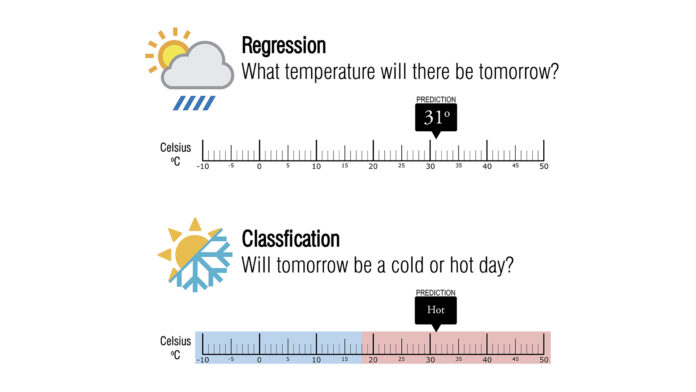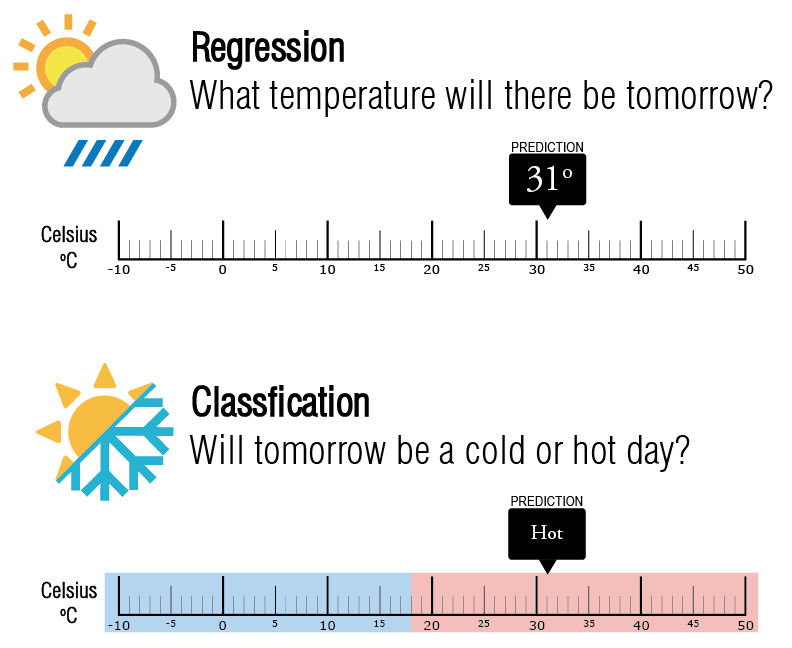# What is the difference between regression and classification in Machine Learning?

0
10In the field of Machine Learning, the abundance of terms and techniques can be overwhelming, and at times, different concepts may appear similar. Two terms that are often confused but are crucial to understand separately are “regression” and “classification.”

Machine Learning integrates both techniques, but they are applied in different situations and pursue different objectives. Understanding these differences is crucial for selecting the appropriate technique for each problem and thus optimizing the results obtained.

In this article, we will focus on clarifying the differences between regression and classification, explore their specific applications, and discuss how and when to use each of these techniques in Machine Learning projects.

##### Basic Concepts

The image shows a graphical representation illustrating the difference between classification and regression. A regression algorithm in Machine Learning aims to estimate numerical values and develop a mathematical model that fits most of the blue points.

This means that, by providing a series of points, the regression algorithm tries to generate a model that traces the red line observed in the image.

On the other hand, classification algorithms aim to separate a dataset into two or more categories, hence the term “classification.” In the example in the image, two classes are presented: purple circles and yellow squares. The goal of the classification model is to differentiate the data and assign each sample to the category that the algorithm deems relevant.

The following image shows a real-world example illustrating the differences in the use of a regression and a classification algorithm.Let’s imagine we have two algorithms: one for classification and one for regression. These have been trained to predict the weather for the next day, specifically the temperature. The regression algorithm will provide a specific temperature value, while the classifier will simply indicate whether the day will be cold or warm.

The classifier is responsible for categorizing a set of data into specific classes. On the other hand, the regressor aims to estimate a specific numerical value.

##### Applications of Regression Algorithms
1. Real Estate Price Prediction: A regression model can be used to predict the price of a house based on its features, such as size, number of rooms, location, etc.

2. Income Prediction: Regression can be used to predict the future income of an individual or a company based on variables like age, education, and work experience.
3. Time Series Analysis: Regression is useful for predicting future values in time series data, such as forecasting stock prices, quarterly sales, or product demand over time.

4. Air Quality Prediction: Regression models can predict air quality based on variables like pollutant concentration, temperature, and humidity.

5. Estimating Age of Individuals: Regression can be used to estimate a person’s age based on facial features, as in facial recognition applications.

##### Applications of Classification Algorithms
1. Spam Detection: Spam filters use classification algorithms to determine whether an email is spam or non-spam based on its content and message characteristics.

2. Medical Diagnosis: Classification models can assist in medical diagnosis, such as disease detection based on medical test results or medical images.
3. Document Classification: Classification is used to organize documents into categories, such as news, emails, reports, etc., in document management applications.
4. Fraud Detection in Financial Transactions: Classification algorithms can identify fraudulent transactions based on behavioral patterns and transaction characteristics.
5. Image Classification: In computer vision applications, like object recognition, classification is used to label objects in images.
6. Sentiment Analysis on Social Media: Classification models can determine whether a comment on a social network is positive, negative, or neutral, which is useful for sentiment analysis.

##### Conclusion

In summary, in the field of Machine Learning, it is essential to understand the differences between regression and classification, two fundamental techniques in supervised learning.

Regression is used to estimate numerical values, while classification is employed to categorize data into different classes. These techniques have diverse applications and are selected based on the type of problem at hand. Whether predicting real estate prices or detecting spam in emails, choosing the right technique is crucial for the success of a Machine Learning project.

By understanding these differences and applications, professionals can make informed decisions to effectively address problems and achieve accurate results.